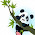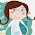Thursday, March 20, 2014

Easter Math: A Fun Way to Work on Combinations of 5 and 10

If you have been reading my posts lately, you might have noticed I have been talking a lot about combinations of 5, 10, 20 and 100!  These are such important skills for kids to be fluent with and we are heading into that time of year where fluency with these ideas really matters.

Today I want to share with you a quick and easy way to give students another chance to practice these combinations but still have fun!  All you need is some plastic Easter eggs and jelly beans or small candies.  (I used these Starburst jelly beans.  They are so much tastier than regular jelly beans.  They are also slightly smaller so you can fit more of them under an egg.

Here are the Common Core standards addressed by this activity
KINDERGARTEN
CCSS.MATH.CONTENT.K.OA.A.4
For any number from 1 to 9, find the number that makes 10 when added to the given number, e.g., by using objects or drawings, and record the answer with a drawing or equation.

CCSS.MATH.CONTENT.K.OA.A.5
Fluently add and subtract within 5FIRST GRADE
CCSS.MATH.CONTENT.1.OA.C.6
Add and subtract within 20, demonstrating fluency for addition and subtraction within 10. Use strategies such as counting on; making ten (e.g., 8 + 6 = 8 + 2 + 4 = 10 + 4 = 14); decomposing a number leading to a ten (e.g., 13 - 4 = 13 - 3 - 1 = 10 - 1 = 9); using the relationship between addition and subtraction (e.g., knowing that 8 + 4 = 12, one knows 12 - 8 = 4); and creating equivalent but easier or known sums (e.g., adding 6 + 7 by creating the known equivalent 6 + 6 + 1 = 12 + 1 = 13).
CCSS.MATH.CONTENT.1.OA.D.8
Determine the unknown whole number in an addition or subtraction equation relating three whole numbers. For example, determine the unknown number that makes the equation true in each of the equations 8 + ? = 11, 5 = _ - 3, 6 + 6 = _.

CCSS.MATH.CONTENT.2.OA.B.2
Fluently add and subtract within 20 using mental strategies.2 By end of Grade 2, know from memory all sums of two one-digit numbers.

Combinations of 5Put 5 jelly beans on the table.  Using one part of a plastic Easter egg, cover up some of the jelly beans.  You can have kids play this game in pairs and have one kid look away while the other covers some of the jelly beans.  Then they have to figure out how many jelly beans are covered up.  In this case, what goes with 3 to make 5?Have kids keep a simple record sheet and record equations.  You can have them use addition or subtraction equations depending on where your class is comfortable or where they need practice.Once kids are fluent with combinations to 5, have them play with 10 jelly beans.  You can also choose other numbers because it is great practice decomposing any number into other parts!The record sheet can simply be done on dry erase boards or scrap paper.
Looking for other ways to practice combinations of numbers?  Check out these ideas
Here is a game where you just need 2 color counters or pennies.  NO PREP!
Here is how to extend the last game for combinations of 20
Here is a bunch of quick and easy ways I work on combinations of 100

Next week I will be following up this work on combinations of 10 with this great take away from 10 number puzzle.Here is one of 10 new number puzzles.  This one is a great follow up to a lesson on combinations of 10!  They are self checking because they won't fit together if kids get the wrong answer.  The 10 frame is there to support kids who still need a visual image.  I will follow this up with some formative assessment around subtracting from 10.

Do you have any good ideas for practicing combinations of numbers?  Please feel free to share ideas or links in the comment section below!

1.Such a fun way to use those Easter eggs. Thank you!

1.This is one of the most fun ways to practice a very important skill!

2.I love this idea! What a fun way to reinforce this concept. We have been working very hard with missing addend, and I think this will be a perfect addition to our small group practice. Thanks so much :) Excited to be following you!

Kelly
1.